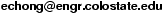# An Introduction to Optimization

## Contents

Preface

### Part I. Mathematical Review

1 Methods of Proof and Some Notation
1.1 Methods of Proof
1.2 Notation
2 Real Vector Spaces and Matrices
2.1 Real Vector Spaces
2.2 Rank of a Matrix
2.3 Linear Equations
2.4 Inner Products and Norms
3 Transformations
3.1 Linear Transformations
3.2 Eigenvalues and Eigenvectors
3.3 Orthogonal Projections
3.4 Quadratic Forms
3.5 Matrix Norms
4 Concepts from Geometry
4.1 Line Segments
4.2 Hyperplanes and Linear Varieties
4.3 Convex Sets
4.4 Neighborhoods
4.5 Polytopes and Polyhedra
5 Elements of Differential Calculus
5.1 Differentiability
5.2 The Derivative Matrix
5.3 Chain Rule
5.4 Level Sets and Gradients
5.5 Taylor Series

### Part II. Unconstrained Optimization

6 Basics of Unconstrained Optimization
6.1 Introduction
6.2 Conditions for Local Minima
7 One-Dimensional Search Methods
7.1 Golden Section Search
7.2 Fibonacci Search
7.3 Newton's Method
7.4 Secant Method
7.5 Remarks on Line Search Methods
8 Gradient Methods
8.1 Introduction
8.2 The Method of Steepest Descent
8.3 Analysis of Gradient Methods
9 Newton's Method
9.1 Introduction
9.2 Analysis of Newton's Method
10 Conjugate Direction Methods
10.1 Introduction
10.2 The Conjugate Direction Algorithm
10.3 The Conjugate Gradient Algorithm
10.4 The Conjugate Gradient Algorithm for Non-Quadratic Problems
11 Quasi-Newton Methods
11.1 Introduction
11.2 Approximating the Inverse Hessian
11.3 The Rank One Correction Formula
11.4 The DFP Algorithm
11.5 The BFGS Algorithm
12 Solving Ax=b
12.1 Least-Squares Analysis
12.2 Recursive Least-Squares Algorithm
12.3 Solution to Ax=b minimizing ||x||
12.4 Kaczmarz's Algorithm
12.5 Solving Ax=b in General
13 Unconstrained Optimization and Feedforward Neural Networks
13.1 Introduction
13.2 Single Neuron Training
13.3 The Backpropagation Algorithm
14 Genetic Algorithms
14.1 Basic Description
14.2 Analysis of Genetic Algorithms

### Part III. Linear Programming

15 Introduction to Linear Programming
15.1 A Brief History of Linear Programming
15.2 Simple Examples of Linear Programs
15.3 Two-Dimensional Linear Programs
15.4 Convex Polyhedra and Linear Programming
15.5 Standard Form Linear Programs
15.6 Basic Solutions
15.7 Properties of Basic Solutions
15.8 A Geometric View of Linear Programs
16 The Simplex Method
16.1 Solving Linear Equations Using Row Operations
16.2 The Canonical Augmented Matrix
16.3 Updating the Augmented Matrix
16.4 The Simplex Algorithm
16.5 Matrix Form of the Simplex Method
16.6 The Two-Phase Simplex Method
16.7 The Revised Simplex Method
17 Duality
17.1 Dual Linear Programs
17.2 Properties of Dual Problems
18 Non-Simplex Methods
18.1 Introduction
18.2 Khachiyan's Algorithm
18.3 Karmarkar's Algorithm

### Part IV. Nonlinear Constrained Optimization

19 Problems with Equality Constraints
19.1 Introduction
19.2 Problem Formulation
19.3 Tangent and Normal Spaces
19.4 Lagrange Conditions
19.5 Second-Order Conditions
19.6 Minimizing ||x|| Subject to Ax=b: A Lagrange Perspective
20 Problems With Inequality Constraints
20.1 Karush-Kuhn-Tucker Conditions
20.2 Second-Order Conditions
21 Convex Optimization Problems
21.1 Introduction
21.2 Convex Functions
21.3 Optimization of Convex Functions
22 Algorithms for Constrained Optimization
22.1 Projected Gradient Methods
22.2 Penalty Methods
Bibliography
Index

Professor Edwin K. P. Chong,This page was last modified .